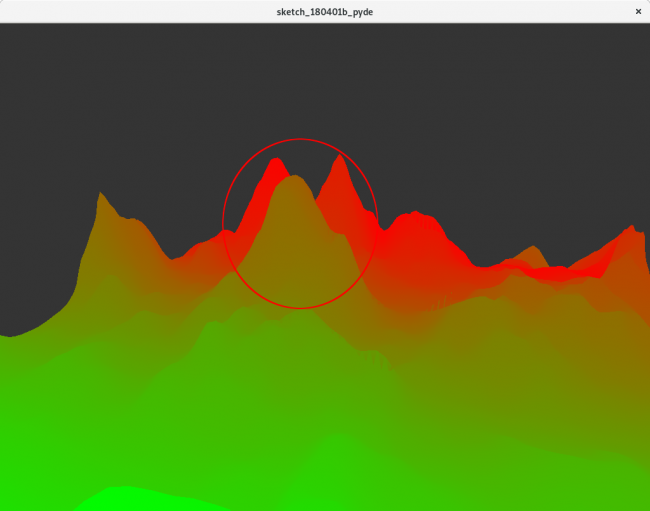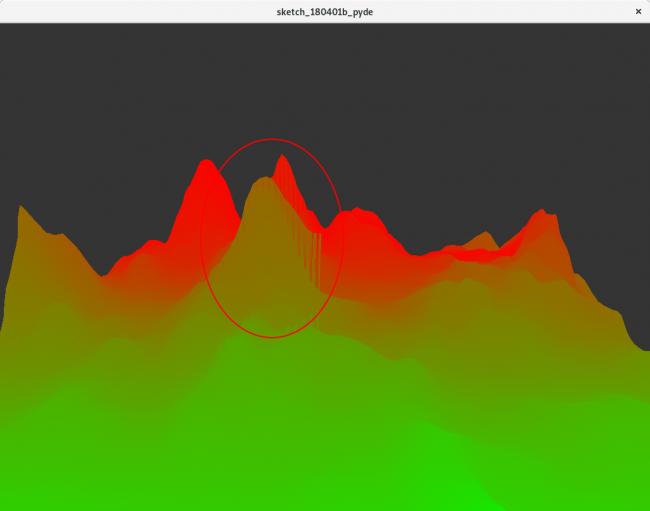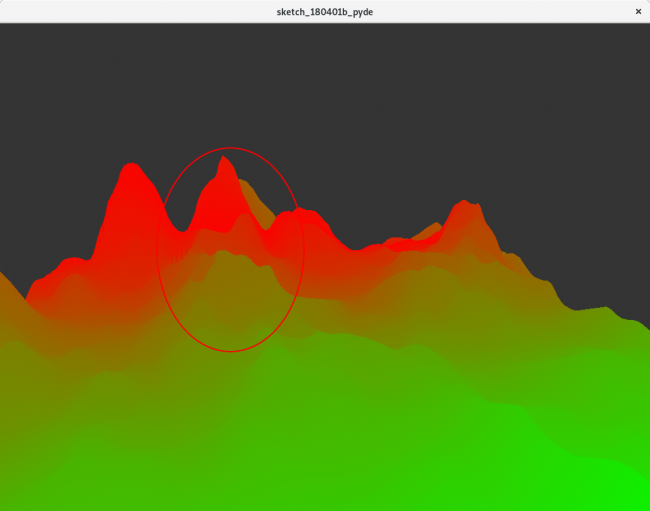#### Howdy, Stranger!

We are about to switch to a new forum software. Until then we have removed the registration on this forum.

# processing.py Z-Buffer problems when drawing triangle mesh

edited April 2018

Hi folks,

I'm currently trying around with terrain generation using perlin noise. Therefore, I generate a map of 3d vertices in a shape and render the shape with a texture (see the below code).

However, when rotating the map, it seems like parts of the mesh are rendered in wrong order, since there are artefacts and overlaps. See the following three images for the same szene, rotated by a few degrees.I really think its a problem due to my code (it has been ages since I last did some real 3d coding), for what its worth: I'm running this code on a arch linux system (kernel 4.15.14-1-ARCH) and I've got a integrated intel hd graphics card.

The code I use to render this scene is the following (I skipped some parts for brevity): edit: I've added the parts missing for letting the example run. Thanks to jeremydouglass for the comment!

``````scale = 20
zangle = 0
l_width = 100
l_height = 100

def setup():
size(1024, 768, P3D)
background(52)

def calc_elevation(w, h, octaves=4):
elevation = []

for y in range(0, h):
column = []
for x in range(0, w):
nx = map(x, 0, w, 0, 1)
ny = map(y, 0, h, 0, 1)
frequency = 1
hight_value = 0
for _ in range(octaves):
hight_value += 1 / frequency * noise(5 * nx * frequency, 5 * ny * frequency)
frequency *= 2
hight_value = pow(hight_value, 3.0)
column.append(hight_value)
elevation.append(column)
return elevation

def keyPressed():
global zangle
if key == 'a':
zangle += -PI / 10
elif key == 'd':
zangle += PI / 10

def draw():
global zangle

background(52)
textSize(20)
translate(width/2, height/2, -600)
rotateX(PI / 2 * 0.8)
rotateZ(zangle)

elevation = calc_elevation(l_width, l_height, 2)
max_height = max([max(list) for list in elevation])

noStroke()
mesh = createShape()

img = createImage(100, 100, RGB)
for y in range(100):
for x in range(100):
img.pixels[y * 100 + x] = color((float(x) / float(100)) * 255, (1 - (float(x) / float(100))) * 255, 0)

mesh.setTexture(img)
mesh.beginShape(TRIANGLE)
for y in range(-l_height / 2, l_width / 2 - 1):
for x in range(-l_width / 2, l_width / 2 - 1):
mesh.vertex((x + 1) * scale,
y * scale,
(elevation[y + l_height / 2][x + 1 + l_width / 2] - (max_height / 2)) * scale * 50,
map(x + 1, -l_width / 2, l_width / 2, 0, img.width),
map(y, -l_height / 2, l_height / 2, 0, img.height)
)
mesh.vertex(x * scale,
y * scale,
(elevation[y + l_height / 2][x + l_width / 2] - (max_height / 2)) * scale * 50,
map(x, -l_width / 2, l_width / 2, 0, img.width),
map(y, -l_height / 2, l_height / 2, 0, img.height)
)
mesh.vertex(x * scale,
(y + 1) * scale,
(elevation[y + 1 + l_height / 2][x + l_width / 2] - (max_height / 2)) * scale * 50,
map(x, -l_width / 2, l_width / 2, 0, img.width),
map(y + 1, -l_height / 2, l_height / 2, 0, img.height)
)

mesh.vertex((x + 1) * scale,
y * scale,
(elevation[y + l_height / 2][x + 1 + l_width / 2] - (max_height / 2)) * scale * 50,
map(x + 1, -l_width / 2, l_width / 2, 0, img.width),
map(y, -l_height / 2, l_height / 2, 0, img.height)
)
mesh.vertex(x * scale,
(y + 1) * scale,
(elevation[y + 1 + l_height / 2][x + l_width / 2] - (max_height / 2)) * scale * 50,
map(x, -l_width / 2, l_width / 2, 0, img.width),
map(y + 1, -l_height / 2, l_height / 2, 0, img.height)
)
mesh.vertex((x + 1) * scale,
(y + 1) * scale,
(elevation[y + 1 + l_height / 2][x + 1 + l_width / 2] - (max_height / 2)) * scale * 50,
map(x + 1, -l_width / 2, l_width / 2, 0, img.width),
map(y + 1, -l_height / 2, l_height / 2, 0, img.height)
)
mesh.endShape(TRIANGLE)
shape(mesh, 0, 0)
``````

edit: If removing the "noStroke" option, the problem becomes even more obvious. It seems as if some of the vertices are rendered on top of others...

Tagged:

• A runnable example (MCVE) without code errors is much better.

``````img.pixels[y * 100 + x] = color((x_val / 100.0)) * 255, (1 - (x_val / 100.0)) * 255, 0)
``````

mismatched input ')' expecting NEWLINE

``````translate(width/2, height/2, ztranslate)
``````

global name 'ztranslate' is not defined

et cetera.

I might suggest trying to enable the depth test hint -- but can't try it, since this isn't a working example.

• Thanks for your suggestion, see above for the updated excerp! I tried to find a more simple calculation for the hight map to reduce the code length, however, i was to uncreative …

• Hmm. Have you tried running what you pasted to the forum?

``````            img.pixels[y * 100 + x] = color((x_val / 100.0)) * 255, (1 - (x_val / 100.0)) * 255, 0)
``````

mismatched input ')' expecting NEWLINE

I can clearly see 4 ( and 5 ) in that line. That just isn't right.

• Aah… I must have pasted the wrong code with my last update, thanks for the comment and patience…

• Great -- it renders! But I can't see the bug you showed above. Should there be rotation code to the keys or mouse...?

• The rendered image should rotate 10 degree-wise, using the a & d keys (lines 28-33).